胡晋红张晓乐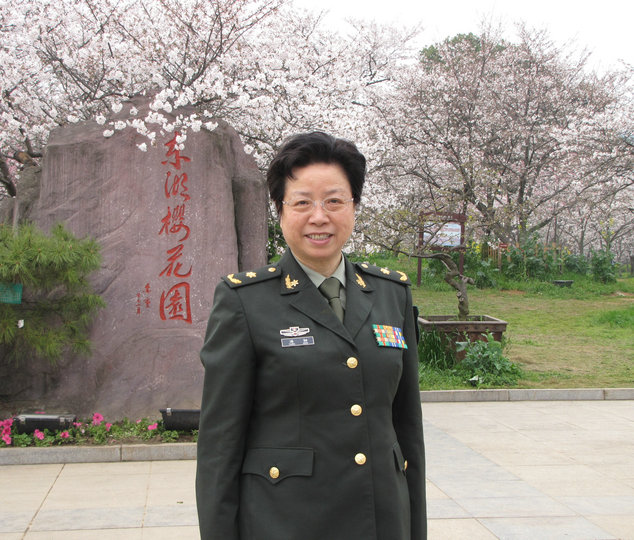汤韧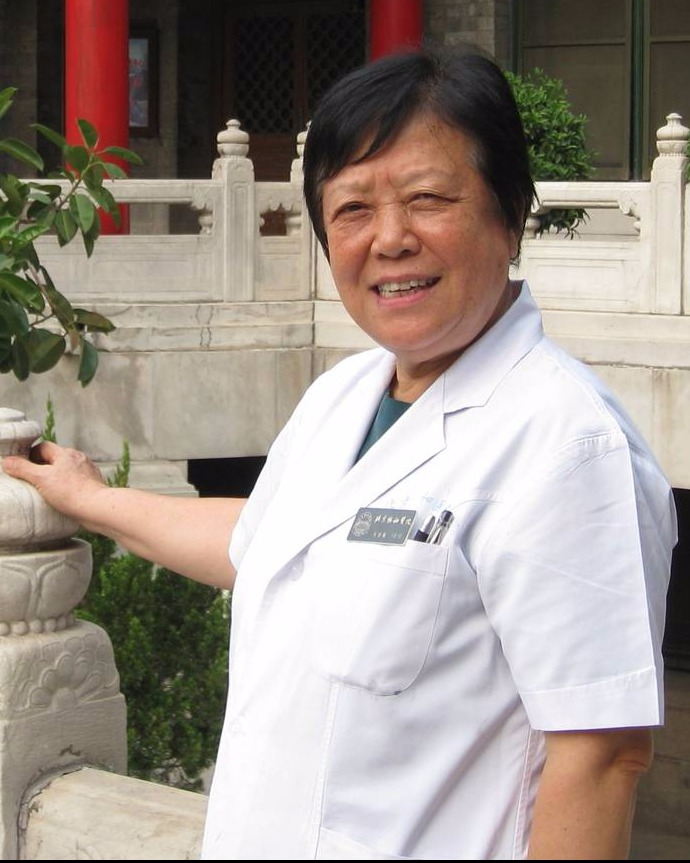张继春刘治军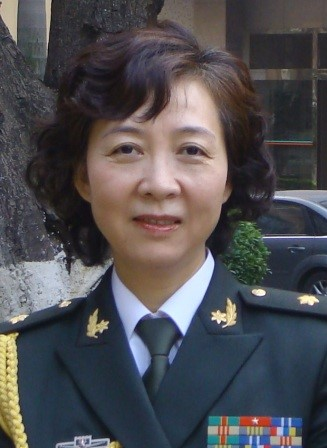吴新荣张翠莲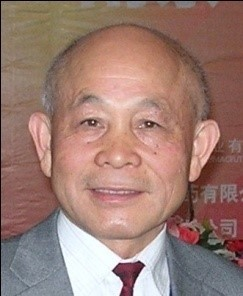袁锁中张红敏卢琴郭梦林杨希殷艳刘珺杜伟向春艳王海莲宋伍温渊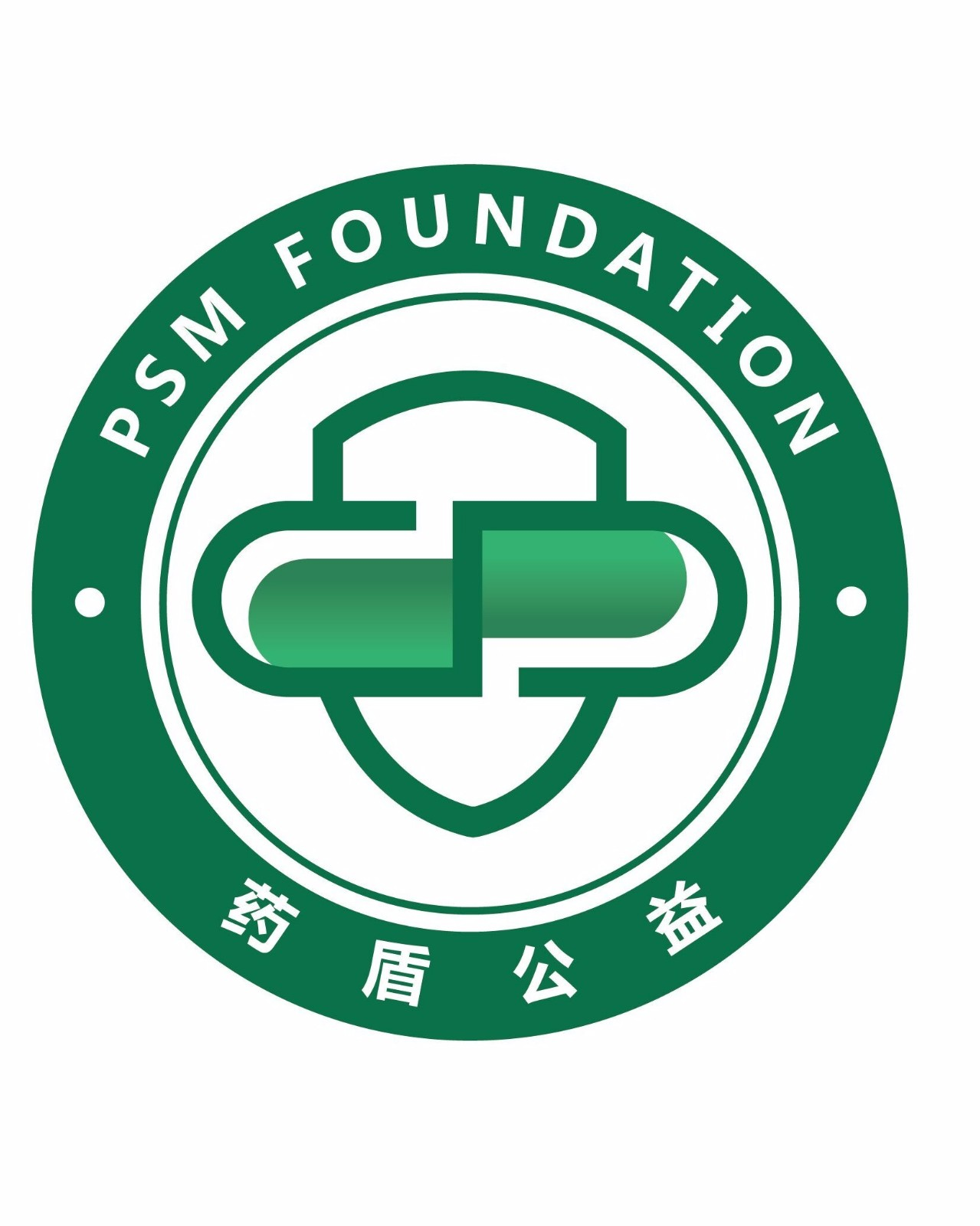薛阳晖温国兴余文浩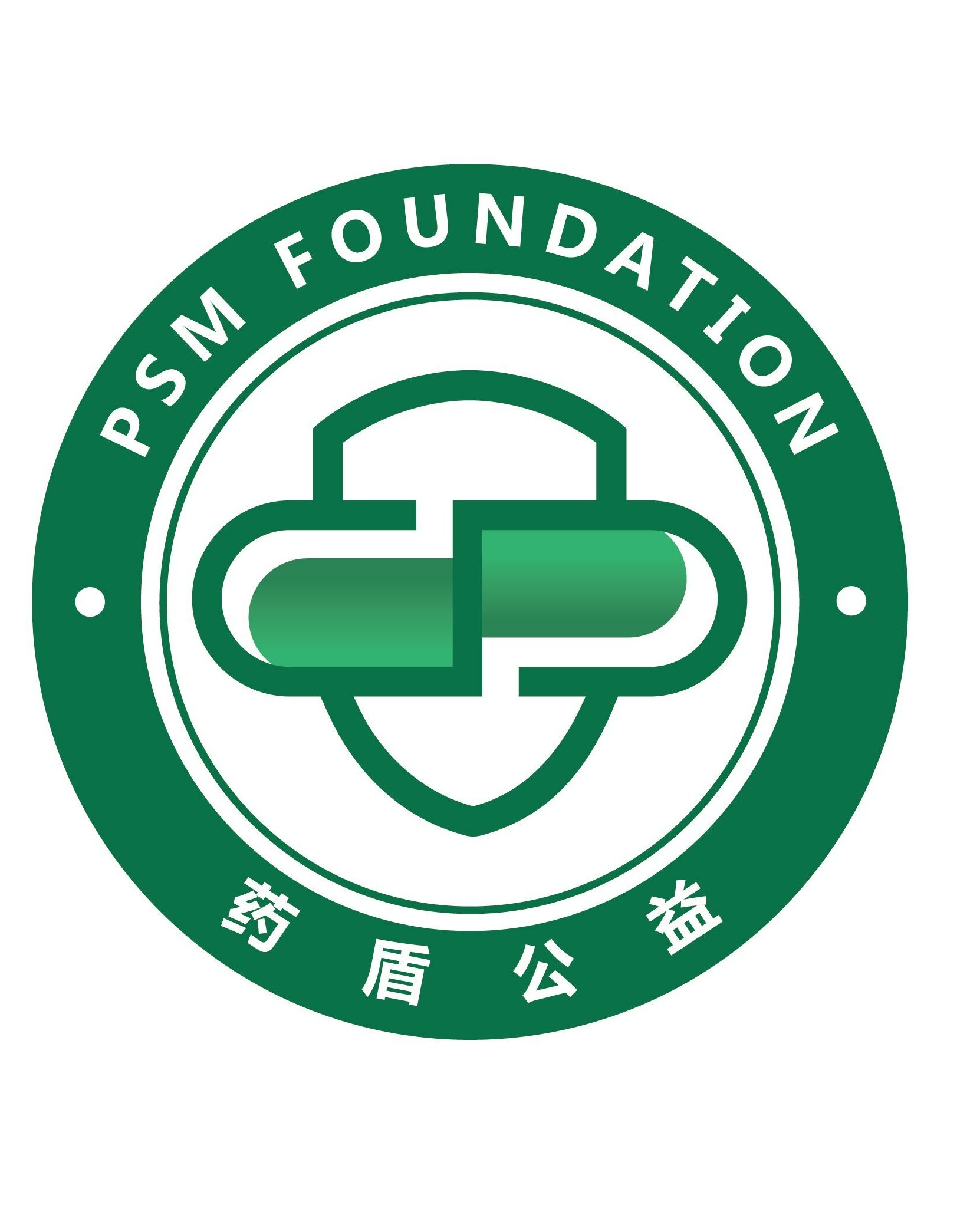朱雪薇

PSM药品安全联盟• （17）
• （0）
• （39）
• （32）
• （37）
• （52）
• （25）
• （0）
• （0）
• （1）
• （49）
• （51）
• （37）
• （39）
• （39）
• （1）
• （9）
• （0）
• （0）
• （1）
• （14）
• （26）
• （0）
• （19）
• （80）
• （1）
• （52）
• （45）
• （39）
• （35）
• （0）
• （7）
• （14）
• （45）
• （8）
• （21）
• （36）
• （30）
• （24）
• （53）
• （15）
• （27）
• （12）
• （38）
• （13）
• （16）
• （3）
• （14）
• （26）
• （0）
• （5）
• （54）
• （0）
• （25）
• （4）
• （1）
• （3）
• （3）